## Expanding Cubed Binomials: Algebra 2/Trig.

Greetings math friends! This post will go over expanding cubed binomials using two different methods to get the same answer. We’re so used to seeing squared binomials such as,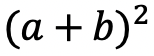, and expanding them without a second thought.  But what happens when our reliable squared binomials are now raised to the third power, such as,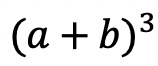?  Luckily for us, there is a Rule we can use:

But where did this rule come from?  And how can we so blindly trust it? In this post we will prove why the above rule works for expanding cubed binomials using 2 different methods:

Why bother? Proving this rule will allow us to expand and simplify any cubic binomial given to us in the future! And since we are proving it 2 different ways, you can choose the method that best works for you.

## Method #1: The Box Method

Step 1: First, focus on the left side of the equation by expanding (a+b)3:

Step 2: Now we are going to create our first box, multiplying (a+b)(a+b). Notice we put each term of (a+b) on either side of the box. Then multiplied each term where they meet.

Step 3: Combine like terms ab and ab, then add each term together to get a2+2ab+b2.

Step 4: Multiply (a2+2ab+b2)(a+b) making a bigger box to include each term.

Step 5: Now combine like terms (2a2b and a2b) and (2ab2 and ab2), then add each term together and get our answer: a3+3a2b+3ab2+b3.

## Method #2: The Distribution Method

Let’s expand the cubed binomial using the distribution method step by step below:

Now that we’ve gone over 2 different methods of cubic binomial expansion, try the following practice questions on your own using your favorite method!

Practice Questions: Expand and simplify the following.

Solutions:

Still, got questions?  No problem! Check out the video above or comment below! Happy calculating! 🙂

**Bonus: Test your skills with this Regents question on!

## How to Graph Equation of a Line, y=mx+b: Algebra

Hi everyone, welcome back to Mathsux! This week we’ll be reviewing how to graph an equation of a line in y=mx+b form. And if you have not checked out the video below, please do! Happy calculating! 🙂

An equation of a line can be represented by the following formula:

y=mx+b

Y-Intercept: This is represented by b, the stand-alone number in y=mx+b. This represents where the line hits the y-axis.  This is always the first point you want to start with when graphing at coordinate point (0,b).

Slope: This is represented by m, the number next to x in y=mx+b. Slope tells us how much we go up or down the y-axis and left or right on the x- axis in fraction form:

Now let’s check out an Example!

Graph the following: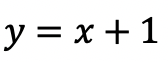-> First, let’s identify the slope and y-intercept of our line.

-> To start, let’s graph the first point on our graph, the y-intercept at point (0,1):

-> Now for the slope. We are going to go up one and over to the right one for each point, since our slope is 1/1.

-> Connect all of our coordinate points and label our graph.

Try the following practice questions on your own!

Practice Questions:

Want more Mathsux?  Don’t forget to check out our Youtube channel and more below! And if you have any questions, please don’t hesitate to comment below. Happy Calculating! 🙂

Need to brush up on slope? Click here to see how to find the rate of change.

## 4 Ways to Factor Trinomials: Algebra

Greeting math peeps and welcome to MathSux! In this post, we are going to go over 4 ways to Factor Trinomials and get the same answer, including, (1) Quadratic Formula (2) Product/Sum, (3) Completing the Square, and (4) Graphing on a Calculator.  If you’re looking for more don’t forget to check out the video and practice questions below.  Happy Calculating! 🙂

Also, if need a review on Factor by Grouping or Difference of Two Squares (DOTS) check out the hyperlinks here!

*If you haven’t done so, check out the video that goes over this exact problem, and don’t forget to subscribe!

We will take this step by step, showing 4 ways to factor trinomials, getting the same answer each and every time! Let’s get to it!

## 4 Ways to Factor Trinomials

____________________________________________________________________

## (2) Product/Sum: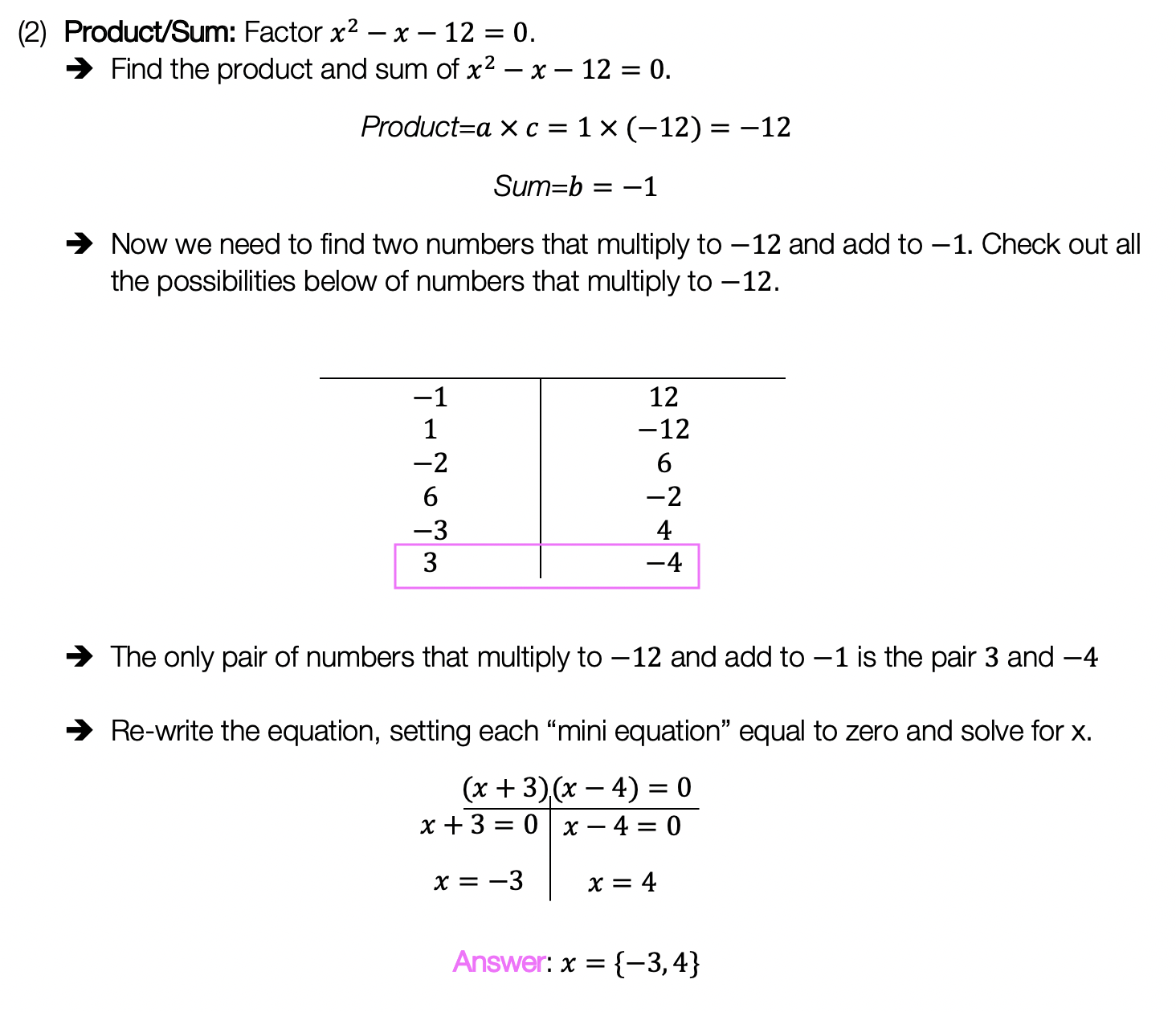____________________________________________________________________

## (3) Completing the Square: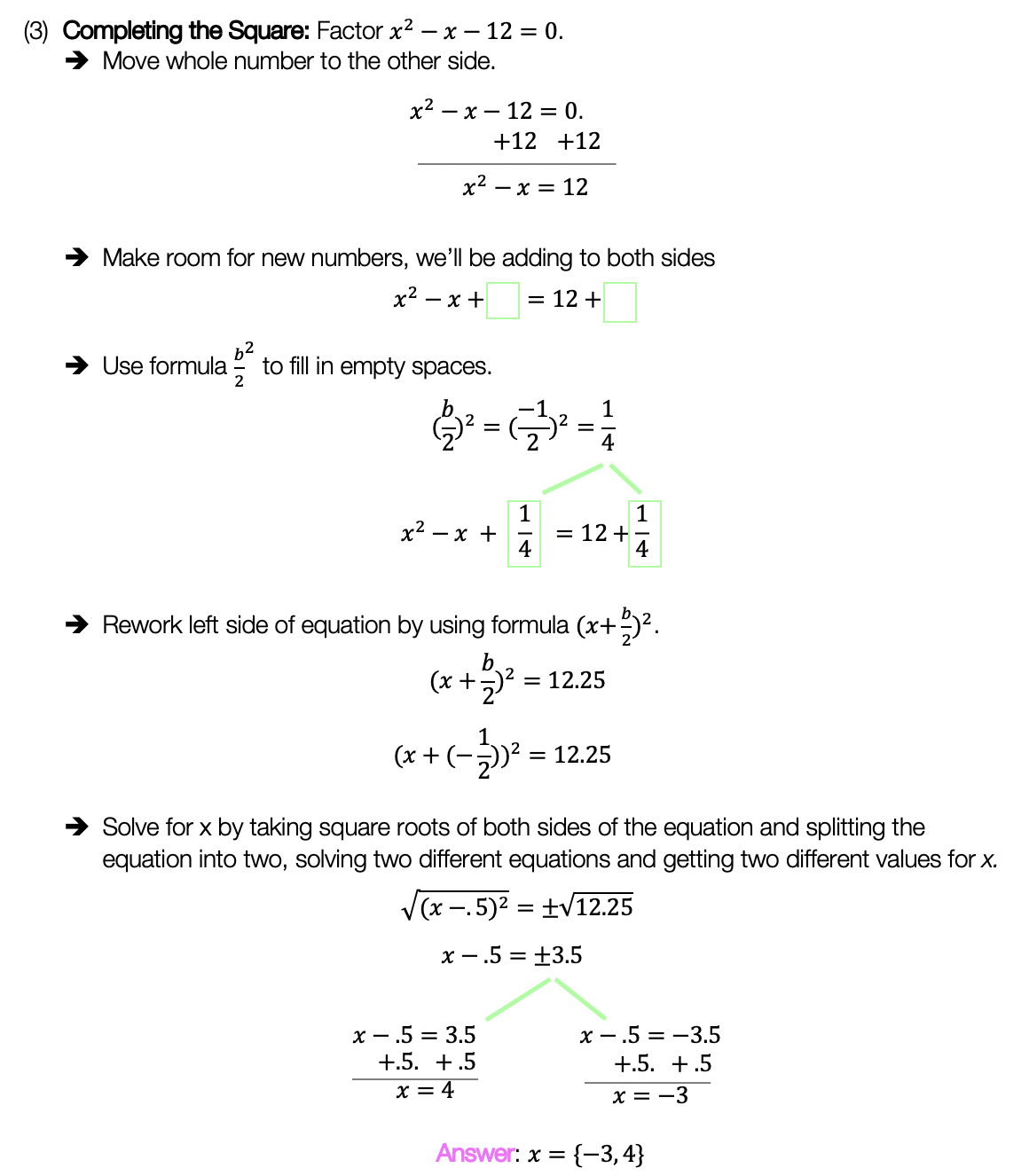____________________________________________________________________

## (4) Graph:

Choose the factoring method that works best for you and try the practice problems on your own below!

Practice Questions:

Solutions:

Want a review of all the different factoring methods out there?  Check out the ones left out here (DOTS and GCF) and happy calculating! 🙂

For even more ways to factor quadratic equations, check out How to factor by Grouping here! 🙂

Also, if you want more Mathsux?  Don’t forget to check out our Youtube channel and more below! If you have any questions, please don’t hesitate to comment below. Happy Calculating! 🙂

## Median of a Trapezoid Theorem: Geometry

Hi everyone and welcome to Math Sux! In this post, we are going to look at how to use and applythe median of a trapezoid theorem. Thankfully, it is not a scary formula, and one we can easily master with a dose of algebra. The only hard part remaining, is remembering this thing! Take a look below to see a step by step tutorial on how to use the median of a trapezoid theorem and check out the practice questions at the end of this post to truly master the topic. Happy calculating! 🙂

*If you haven’t done so, check out the video that goes over this exact problem, also please don’t forget to subscribe!

Step 1:  Let’s apply the Median of a Trapezoid Theorem to this question!  A little rusty?  No problem, check out the Theorem below.

Median of a Trapezoid Theorem: The median of a trapezoid is equal to the sum of both bases.Step 2: Now that we found the value of x , we can plug it back into the equation for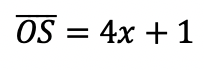median,  to find the value of median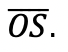Want more practice?  Your wish is my command! Check out the practice problems below:

Practice Questions:

1.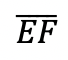is the median of trapezoid ABCDEF, find the value of the median, given the following:2.is the median of trapezoid ACTIVE, find the value of the median, given the following:3.is the median of  trapezoid DRAGON, find the value of the median, given the following:

4.is the median of trapezoid MATRIX, find the value of the median, given the following:

Solutions:

Need more of an explanation?  Check out the detailedand practice problems. Happy calculating! 🙂

## Dividing Polynomials: Algebra 2/Trig.

Greeting math peeps! In this post we are going to go over dividing polynomials! At some point, you may need to know how to answer these types of questions. The cool thing about dividing polynomials is that it’s the same long division you did way back in grade school (except now with a lot of x). Ok, let’s get to it and check out the question below:

Also, if you haven’t done so, check out the video related that corresponds to this problem on Youtube below! 🙂

Explanation:

How do I answer this question?

The question wants us to divide polynomials by using long division.

How do we do this?

Step 1: First we set up a good ole’ division problem with the divisor, dividend, and quotient to solve.

Step 2: Now we use long division like we used to back in the day! If you have any confusion about this please check out the video in this post.

## What if there’s a Remainder?

What happens when there is a remainder though!?!? When dividing polynomials with a remainder in the quotient, the answer is found and checked in a very similar way! Check it out in the example below:

Notice we represented the remainder by adding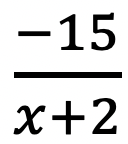to our quotient! We just put the remainder over the divisor to represent this extra bit of solution.

Want more practice? Try solving these next few examples on your own.

## Solutions:

If you’re looking for more on dividing polynomials, check out this post on synthetic division and finding zeros here!

Still got questions? No problem! Don’t hesitate to comment with any questions or check out the video above. Happy calculating! 🙂

## Earth Day Fractals!

In honor of Earth Day last week, I thought we’d take a look at some math that appears magically in nature.  Math? In nature?  For those of you who think math is unnatural or just terrible in general, this is a great time to be proven otherwise!

The key that links math to nature is all about PATTERNS. All math is based on is patterns.  This includes all types of math, from sequences to finding x, each mathematical procedure follows some type of pattern. Meanwhile back in the nearest forest, patterns are occurring everywhere in nature.

The rock star of all patterns would have to be FRACTALS. A Fractal is a repeating pattern that is ongoing and has different sizes of the exact same thing!  And the amazing thing is that we can actually find fractals in our neighbor’s local garden.

Let’s look at some Fractal Examples:

(1) Romanesco Broccoli:  Check out those repeating shapes, that have the same repeating shapes on those shapes and the same repeating shapes on even smaller shapes and…. my brain hurts!

######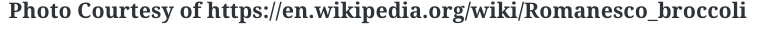(2) Fern Leaves:  The largest part of this fractal is the entire fern leaf itself.  The next smaller and identical part is each individual “leaf” along the stem.  If you look closely, the pattern continues!

######(3) Leaves:  If you’ve ever gotten up real close to any type of leaf, you may have noticed the forever repeating pattern that gets smaller and smaller. Behold the power and fractal pattern of this mighty leaf below!

######.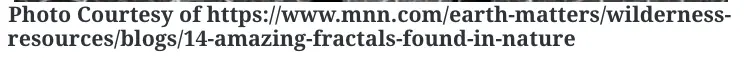Just in case fractals are still a bit hard to grasp, check out the most famous Fractal below,  otherwise known as Sierpinski’s Triangle.  This example might not be found in your local back yard, but it’s the best way to see what a fractal truly is up close and infinite and stuff.

Looking for more math in nature?  Check out this post on the Golden Ratio and happy calculating! 🙂

|    |

## Perpendicular Lines through a Given Point: Geometry

Ahoy math peeps! I’m writing this during the time of the coronavirus, and although, the NYS Regents tests may be canceled, online zooming is still on! From the good ole’ days of test-taking and sitting in a giant room together, I bring to you a Regent’s classic, a question about how to find perpendicular lines through a given point. We will go over the following Regents question, starting with a review of what perpendicular lines are. Stay curious and happy calculating! 🙂

Perpendicular Lines: When two lines going in opposite directions come together to form a perfect 90º angle! Sounds magical, am I right? Check it out for yourself below:

A super exciting feature of these so-called perpendicular lines is that their slopes are negative reciprocals of each other. Wait, what?

How do we do this? Now it is time to go back and answer our question!

First, our equation 2y+3x=1 looks kind of cray, so let’s get it back to normal in y=mx+b form:

Need more of an explanation? Check out the video that goes over these types of questions up on Youtube (video at top of post) and let me know if you have still any questions.

Happy Calculating! 🙂

Looking for more on Perpendicular and Parallel lines? Check out the difference between the two in this post here!

## COVID-19: What does #FlattenTheCurve even mean?

COVID-19: What does #FlattenTheCurve even mean? If you are a human on Earth, then I’m sure you’ve heard about the coronavirus and are currently social distancing. Here in NYC, I’m quarantining like everyone else and listening to all the beautiful math language that has suddenly become mainstream (so, exciting)!  #FlattenTheCurve has become NY’s new catchphrase and for anyone confused about what that means, you’ve come to the right place!

The coronavirus spreads at an Exponential Rate, which means it spreads in a way that increases faster and faster every day.

What does this mean?

For Example, one person with the virus can easily spread the virus to 5 other people, those 5 people can then spread the virus to another 5 people each for a total of an extra 25 people, these 25 people can then spread it to another 5 people each for an extra 125 infected people! And the pattern continues……. See below to get a clearer picture:

.   *Note: These numbers are not based on actual coronavirus data

The Example we just went over is equal to the exponential equation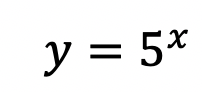, but it is only that, an Example! The exact pattern and exponential equation of the future progress of the virus is unknown! We mathematicians, can only measure what has already occurred and prepare/model for the future.  To make the virus spread less rapidly, it is our duty to stay home to slow the rate of this exponentially spreading virus as much as possible.

We want to #FlattenTheCurve a.k.a flatten the increasing exponential curve of new coronavirus cases that appear every day! Hopefully, this post brings some clarity to what’s going on in the world right now.  Even with mathematics, the true outcome of the virus may be unknown, but understanding why we are all at home in the first place and the positive impact it has is also important (and kind of cool).

Stay safe math friends and happy calculating! 🙂

Want to make math suck just a little bit less? Subscribe and follow us for FREE fun colorful math videos and lessons every week! 🙂

## Math Resources (in the time of COVID)

Calling all students, teachers, and parents!  As everyone is stuck at home during a global pandemic, now is a great time we are all forced to try and understand math (and our sanity level) a little bit more.  Well, I may not be able to help you with the keeping sanity stuff, but as far as math goes, hopefully, the below math resources offer some much needed mathematic support.

All jokes aside I hope everyone is staying safe and successfully social distancing.  Stay well, math friends! 🙂

Kahn Academy: The same Kahn Academy we know and love still has amazing videos and tutorials as usual, but now they also have a live “homeroom” chat on Facebook LIVE every day at 12:00pm. The chats occur daily with Kahn Academy founder Sal and at times feature famous guests such as Bill Gates. Click the link below for more:

Study.com: In a time when companies are being more generous, Study.com is here for us as they offer up to 1000 licenses for school districts and free lessons for teachers, students, and parents.  Check out all the education freebies here:

Study.com

Math PlanetIf you’re looking for free math resources in Pre-Algebra, Algebra, Algebra 2, and Geometry then you will find the answers you need at Math Planet.  All free all the time, find their website here:

MathSux: Clearly, I had to mention MathSux, the very site you are on right now! Check out free math videos, lesson, practice questions, and more for understanding math any way that works for you!

MathSux

What is your favorite educational site?  Let me know in the comments, and stay well! 🙂

## Binomial Cubic Expansion: Algebra 2/Trig.

Hey math friends! In this post, we are going to go over Binomial Cubic Expansion by going step by step! We’ll start by reviewing an old Regents question. Then, to truly master the topic, try the practice problems at the end of this post on your own! And, if you still have questions, don’t hesitate to watch the video or comment below. Thanks for stopping by and happy calculating! 🙂

Also, if you’re looking for more on Binomial Cubic Expansion, check out this post here!

## What are Cubed Binomials?

Binomials are two-termed expressions, and now we are cubing them with a triple exponent! See how to tackle these types of problems with the example below:

How do I answer this question?

We need to do an algebraic proof to see if (a+b)3=a3+b3.

How do we do this?

We set each expression equal to one another, and try to get one side to look like the other by using FOIL and distributing. In this case, we will be expanding (a+b)3 to equal (a+b)(a+b)(a+b).

Extra Tip! Notice that we used something called FOIL to combine (a+b)(a+b).  But what does that even mean? FOIL is an acronym for multiplying the two terms together.  It’s a way to remember to distribute each term to one another.  Take a look below:

Add and combine all like terms together and we get!

_______________________________________________________________________

Keep in touch with MathSux and get FREE math videos, lessons, and practice questions straight to your inbox! Thanks for stopping by and happy calculating! 🙂